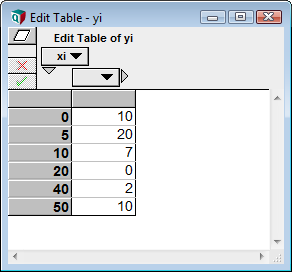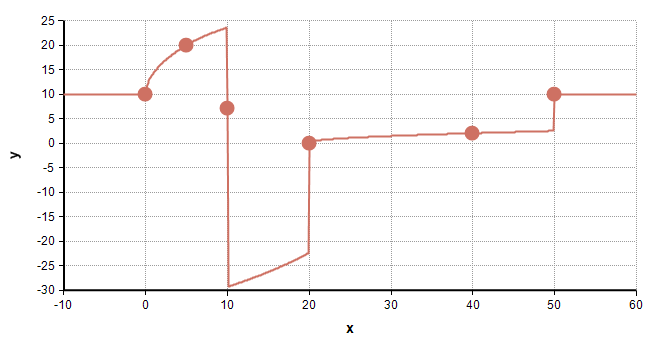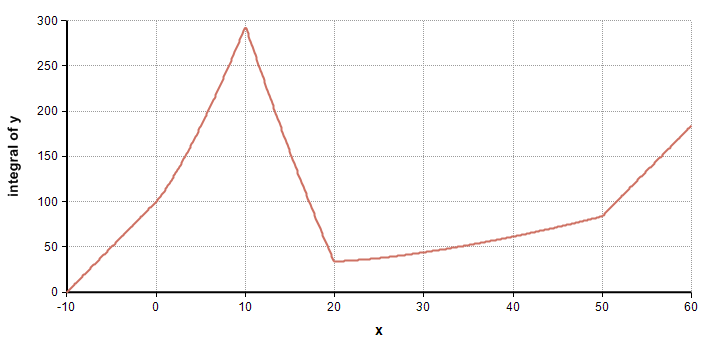# Q SquareInterp

## Q_SquareInterp(xi, yi, x, I )

A function used internally by Analytica to perform an interpolation between points in such a way that an integration of the interpolated curve is linear between the given points. You probably want to consider using LinearInterp or CubicInterp in your own model instead. Q_SquareInterp is not publicly supported, and is not exposed on the Definition menus.

(«xi», «yi») are the given points, and should both be indexed by «I». The index «I» parameter can be omitted if either «xi» or «yi» is an index. The values in «xi» must be strictly increasing. «x» is the value along the x-axis where you want to find the y-value along the interpolated value. Below the smallest «xi» or above the largest «xi», the extrapolated curve is constant.

## Example

The following shows the square-interp for an example set of data points.Index x := Sequence(-10, 60, 0.1)
Q_SquareInterp(xi, yi, x) →The interpolation appears rather odd (which is why this is a not a function that you are likely to use), but the integral of the interpolated curve is piecewise-linear:

Integrate(y, x) →